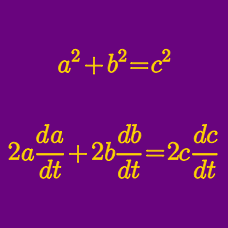Calculus

# Related Rates - Finding Extrema

Eugene is located at $(-12,0)$ and walking east at a rate of $3\text{/sec}.$ Elizabeth is located at $(0,-5)$ and walking north at a rate of $5\text{/sec}.$ What is the largest speed at which they are walking towards each other?

If the relation between $x$ and $y$ is given by $2 x + 8 y = 10,$ what is the maximum value of $3 xy ?$

If the surface area of a cylinder is $294 \pi,$ what is the largest possible volume of the cylinder?

Consider all ordered pairs of real numbers $(x,y)$ which satisfy $x^2 + \frac{y^2}{4} = 1$. What is the maximum value of $8x + \frac{15}{2}y$?

Devin is pumping air into a spherical balloon. If the radius of the balloon is given by $r(t) = -6 t^2 + 23t,$ what is the time $t$ when the volume of the balloon is maximized?

×